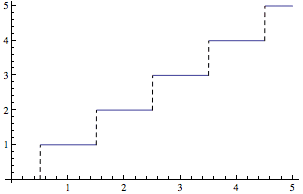# Fourier series of a step function

• B
Hello, can we make a Fourier series expansion of a (increasing or decreasing) step function ? like the one that I attached here. I just want to know the idea of that if it is possible.## Answers and Replies

mfb
Mentor
You can write down the integral, but with an infinite step function like this the integrals will diverge. With a finite step function it works.

•chikou24i
You can write down the integral, but with an infinite step function like this the integrals will diverge. With a finite step function it works.
So you mean that I should take each portion appart (where f(x) is constant) and calculate the integral. Then, I add them all together ?
Is there any other method where I can calculate Fourier series normally and then I multiply them by another factor which will make them increase or decrease ?

mfb
Mentor
The sum will diverge as well for most frequencies, and the approach is at best questionable for frequencies where it does not diverge.

Why do you want to Fourier transform that function?

I want to extract the mathematical function for this graph of voltage response. Could you please help me or guide me in doing that ?
https://fb-s-d-a.akamaihd.net/h-ak-xlp1/v/t1.0-0/p526x296/17457874_1911500359125961_8184758272904545979_n.jpg?oh=50117debf8fd6047caf1f0795e0a9254&oe=59500784&__gda__=1498980987_b71e34fea90ba330474f88d4a58f82d9 [Broken]

Last edited by a moderator:
mfb
Mentor
You could write it as sum of a linear or parabolic function and some periodic term.

I'm not sure if that is really useful, I'm quite sure a piecewise definition of the function will work better.

•chikou24i